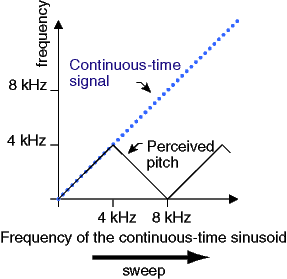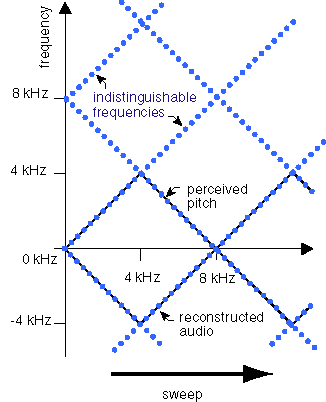EECS20N: Signals and Systems

# Aliasing

In the following applet, you can change the frequency of a "continuous-time" sinusoid from 0 to 8,000 Hz. The sinusoid is sampled at 8 kHz and played through the computer audio system. Notice that as the frequency increases above 4 kHz, the Nyquist frequency, the sound you hear starts to decrease in frequency rather than increase.  Frequencies above 4 kHz are indistinguishable from corresponding frequencies below 4 kHz.  See the text for a mathematical presentation of this phenomenon.

If you were able to run applets, you would have a Aliasing demo here.

If you sweep the frequency of a continuous-time sinusoid from 0 to 8 kHz, and this sinusoid is sampled at 8 kHz and transmitted through the telephone network, the sound you will hear at the other end has a perceived pitch that rises until you get to 4 kHz, but then it begins to fall, as shown in the following plot:Note that in the above applet, you cannot hear the continuous-time signal. But if you could hear it, its perceived pitch would just continue to rise. Instead, your computer reconstructs a continuous-time signal from the sampled signal, and that one has a pitch that does not rise above 4 kHz.

Recall that the frequency-domain representation of a discrete-time signal is periodic with period 2π radians/sample. In radians per second, that is 2π /T, the sampling frequency. In Hertz, it is 1/T. Thus, the sampling frequency is the period of the frequency domain representation of a discrete-time signal. Somehow, the fact that the perceived pitch starts to fall must have something to do with this periodicity.

Recall that the cosine consists of two complex exponentials with frequencies that are negatives of one another (from Euler's relation). The following picture shows the two complex exponentials, and also their periodic repetitions:This picture suggests that what actually happens is simply that the computer hardware chooses from among the periodic frequencies the ones closest to DC. This results in a sinusoid that rises up to 4 kHz, then begins to fall until it drops back down to zero, and then begins to rise again. Indeed, this is exactly what is going on.